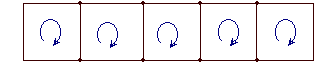# 网格和循环电流方法单击或点击下面的示例电路以调用TINACloud并选择交互式DC模式以在线分析它们。原理图“渔网”或电路图

1. 为每个网格分配网格电流。 尽管方向是任意的，但习惯上使用顺时针方向。

2. 在每个网格周围以与网格电流相同的方向应用基尔霍夫电压定律（KVL）。 如果一个电阻有两个或多个通过它的网格电流，则通过该电阻的总电流将计算为网格电流的代数和。 换句话说，如果流过电阻的电流的方向与环路的网状电流的方向相同，则它的正号为正，否则为负。 像往常一样考虑电压源。如果其方向与网格电流相同，则在KVL方程中将其电压视为正，否则为负。 通常，对于电流源，只有一个网状电流流过该源，并且该电流的方向与该源的电流相同。 如果不是这种情况，请使用本章稍后介绍的更通用的环路电流方法。 对于包含分配给电流源的网格电流的回路，无需编写KVL方程。

3. 求解网格电流的结果循环方程。

4. 使用网格电流确定电路中任何需要的电流或电压。

#### 让我们说明一下 该方法通过以下示例：

-V1 + J.1*（Ri1+R1） - J2*R1 = 0

V2 - J1*R1 + J.2*（R + R1）= 0

-12 + J.1* 17 - J.2* 2 = 0

6 – J1* 2 + J.2* 14 = 0{使用TINA的翻译器解决方案} {Mesh current method} Sys J1，J2 J1*(Ri1+R1)-J2*R1-V1=0 J1*R1+J2*(R1+R)+V2=0 结束; J1 = [666.6667m] J2 = [ - 333.3333m] I：= J1-J2; I =l = b – N + 1

b = Ñ​​-1 + l 因此 l = b – N + 1KVL方程：

J1 *（R1+Ri1）+ J2 * R. i1 - V.1 = 0

-V1+ J1 * Ri1+ J2 *（R + Ri）+ V.2 = 0

Numerically: J1*(15+2)+J2*15-12 = 0

-12 + J1 * 15 + J2 *（15 + 12）+ 6 = 0#### 例如1

IS1.

-V1 + I * R.2 + R.1 *（我–我S1）= 0

#### 数控

I=(10+20*4)/(20+10)=3 A{TINA口译员的解决方案} {使用网格电流方法} 谢谢我 -V1 + I * R2 + R1 *（I – IS1）= 0 结束; I = 

#### 例如2V =（IS3 - 一世S2）* R3=（10-5）* 30 = 150V。您可以使用TINA：进行验证。#### 例如3R1 = R.3 = 100 ohm，R2 = R.4 = 50 ohm，R5 = 20 ohm，R6 = 40 ohm，R7 = 75欧姆。

VS1+I4*（R5+R6+R7） - 一世S*R6 -一世3*（R5 + R.6）= 0

VS2 - 一世3*（R1+R2） - 一世S*R2 +我2*（R1 + R.2）= 0

-VS1 +我3*（R1 + R.2 + R.3 + R.4 + R.5 + R.6）+我S*（R2 +R4 + R.6） - 一世4*（R5 + R.6) - 一世2*（R1 + R.2）= 0

V = R.4 * （一世2 +我3)

100 + I4* 135-2 40 *-I3* 60 = 0

150 + I2* 150-2 50 *-I3* 150 = 0

-100 + I3* 360 2 + 140 *-I4* 60-I2* 150 = 0

V = 50 *（2 + I3)

I4 = D.3/D

– 60 *我3 + 135 * I4= -20

150 * I2-150 * I3 = – 50

-150 * I2+ 360 * I3 - 60 *我4= – 180V = R.4*（2 + I3）= 34.8485 V.

 {使用TINA的翻译器解决方案} 系统I2，I3，I4 Vs2+I2*(R1+R2)-R2*Is-I3*(R1+R2)=0 -Vs1+I3*(R1+R2+R3+R4+R5+R6)+Is*(R2+R4+R6)-I2*(R1+R2)-I4*(R5+R6)=0 Vs1+I4*(R5+R6+R7)-Is*R6-I3*(R5+R6)=0 结束; I2 = [ - 1.6364] I3 = [ - 1.303] I4 = [ - 727.2727m] 五：= R4 *（为+ I3）; V = [34.8485]

X很高兴有你 DesignSoft
如果需要任何帮助找到合适的产品或需要支持，可以进行聊天。